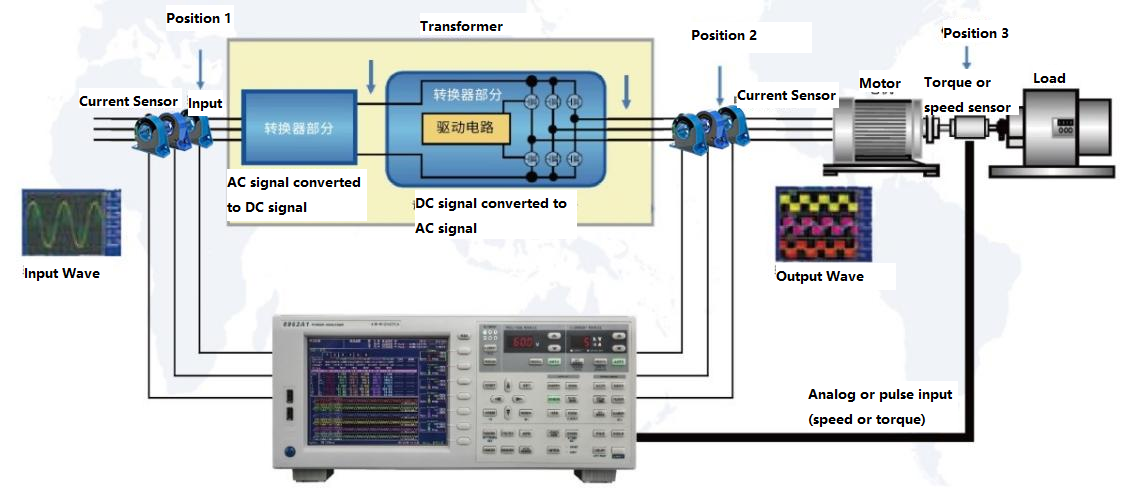Company News

# How to Accurately Measure the Efficiency of Inverter Motors With Current Sensors and Power Meters

Views : 290
Update time : 2022-11-30 11:03:25
As a key part of motor control system and industrial energy saving, frequency converter has the characteristics of high efficiency and energy saving, and has been widely used in various industries. Therefore, the demand for high-precision testing of motor/inverter power consumption efficiency is also increasing. The variable frequency motor efficiency test system is composed of a power meter, a current sensor and a speed sensor, etc. as shown below.The three-phase sinusoidal AC power is inputted to the converter device (that is, the inverter driver), and after being outputted by the converter device, the waveform becomes a pulsating DC waveform to provide power to the motor, and the motor provides power to the load.

The power provided by the power supply at the input end is tested by the instrument, and the power at the output end of the drive is tested at the same time. After the speed torque is obtained through the speed torque sensor, the mechanical efficiency can be calculated.

If you want to calculate the efficiency of the drive, use the front and rear output (position 2) of the drive to compare the input (position 1) power to get the conversion efficiency of the inverter drive. Three current sensors are installed in position 2 and position 1 to accurately measure the current and feed it back to the power meter. If you want to calculate the mechanical efficiency of the motor output, you can use the output (position 3) before and after the speed torque sensor to compare with the input (position 2) to calculate the mechanical energy conversion efficiency of the motor through the formula. In the same way, the efficiency of the entire system can be obtained through the power ratio of the front (position 1) and rear (position 3) of the entire system. The testing difficulties of the entire system is shown as follows:
1 High-precision, wide-band measurement
2 direct input of voltage and current
3 Quickly measure waveforms
4 Simultaneously display parameters such as input voltage, current and power
5 Simultaneously measure the torque and speed of the motor
6 The ratio of input and output at the same time

Error-prone point: Since two meters are used to test the input and output, The inconformity between the two test clocks makes the power to be measured at different time, which causes a huge error in the result.

A six-channel power analyzer with HANGZHI HIT series broadband, high-precision current sensor, can solve the above test problems perfectly.

Related News